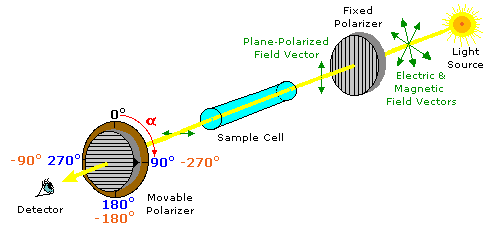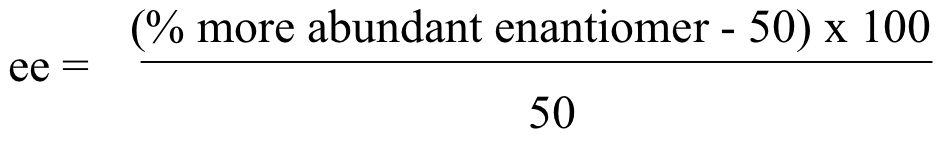# 5.3 Optical Activity

Objectives

After completing this section, you should be able to

1. describe the nature of plane-polarized light.
2. describe the features and operation of a simple polarimeter.
3. calculate the specific rotation of a compound, given the relevant experimental data.

Key Terms

Make certain that you can define, and use in context, the key terms below.

• analyzer
• dextrorotatory
• levorotatory
• optically active
• plane-polarized light
• polarimeter
• polarizer
• specific rotation, $\ce{\sf{[\alpha]_{D}}}$

Study Notes

A polarizer is a device through which only light waves oscillating in a single plane may pass. A polarimeter is an instrument used to determine the angle through which plane-polarized light has been rotated by a given sample. You will have the opportunity to use a polarimeter in the laboratory component of the course. An analyzer is the component of a polarimeter that allows the angle of rotation of plane-polarized light to be determined.

Specific rotations are normally measured at 20°C, and this property may be indicated by the symbol $\ce{\sf{[\alpha]^20_{D}}}$. Sometimes the solvent is specified in parentheses behind the specific rotation value, for example,

$\ce{\sf{[\alpha]^20_{D} = +12}}$° (chloroform)

For liquids, the specific rotation may be obtained using the neat liquid rather than a solution; in such cases the formula is

$[ α ] D temp (neat)= α l×d$

where α is the observed rotation, l is the path length of the cell (measured in decimetres, dm), and d is the density of the liquid.

Identifying and distinguishing enantiomers is inherently difficult, since their physical and chemical properties are largely identical. Fortunately, a nearly two hundred year old discovery by the French physicist Jean-Baptiste Biot has made this task much easier. This discovery disclosed that the right- and left-handed enantiomers of a chiral compound perturb plane-polarized light in opposite ways. This perturbation is unique to chiral molecules, and has been termed optical activity.

## Polarimetry

Plane-polarized light is created by passing ordinary light through a polarizing device, which may be as simple as a lens taken from polarizing sun-glasses. Such devices transmit selectively only that component of a light beam having electrical and magnetic field vectors oscillating in a single plane. The plane of polarization can be determined by an instrument called a polarimeter, shown in the diagram below.Monochromatic (single wavelength) light, is polarized by a fixed polarizer next to the light source. A sample cell holder is located in line with the light beam, followed by a movable polarizer (the analyzer) and an eyepiece through which the light intensity can be observed. In modern instruments an electronic light detector takes the place of the human eye. In the absence of a sample, the light intensity at the detector is at a maximum when the second (movable) polarizer is set parallel to the first polarizer (α = 0º). If the analyzer is turned 90º to the plane of initial polarization, all the light will be blocked from reaching the detector.

Chemists use polarimeters to investigate the influence of compounds (in the sample cell) on plane polarized light. Samples composed only of achiral molecules (e.g. water or hexane), have no effect on the polarized light beam. However, if a single enantiomer is examined (all sample molecules being right-handed, or all being left-handed), the plane of polarization is rotated in either a clockwise (positive) or counter-clockwise (negative) direction, and the analyzer must be turned an appropriate matching angle, α, if full light intensity is to reach the detector. In the above illustration, the sample has rotated the polarization plane clockwise by +90º, and the analyzer has been turned this amount to permit maximum light transmission.

The observed rotations ($$\alpha$$) of enantiomers are opposite in direction. One enantiomer will rotate polarized light in a clockwise direction, termed dextrorotatory or (+), and its mirror-image partner in a counter-clockwise manner, termed levorotatory or (–). The prefixes dextro and levo come from the Latin dexter, meaning right, and laevus, for left, and are abbreviated d and l respectively. If equal quantities of each enantiomer are examined , using the same sample cell, then the magnitude of the rotations will be the same, with one being positive and the other negative. To be absolutely certain whether an observed rotation is positive or negative it is often necessary to make a second measurement using a different amount or concentration of the sample. In the above illustration, for example, α might be –90º or +270º rather than +90º. If the sample concentration is reduced by 10%, then the positive rotation would change to +81º (or +243º) while the negative rotation would change to –81º, and the correct α would be identified unambiguously.

Since it is not always possible to obtain or use samples of exactly the same size, the observed rotation is usually corrected to compensate for variations in sample quantity and cell length. Thus it is common practice to convert the observed rotation, α, to a specific rotation, by the following formula:

$[\alpha]_D = \dfrac{\alpha}{l c} \tag{5.3.1}$

where

• $$[\alpha]_D$$ is the specific rotation
• $$l$$ is the cell length in dm
• $$c$$ is the concentration in g/ml
• $$D$$ designates that the light used is the 589 line from a sodium lamp

Compounds that rotate the plane of polarized light are termed optically active. Each enantiomer of a stereoisomeric pair is optically active and has an equal but opposite-in-sign specific rotation. Specific rotations are useful in that they are experimentally determined constants that characterize and identify pure enantiomers. For example, the lactic acid and carvone enantiomers discussed earlier have the following specific rotations.

 Carvone from caraway: [α]D = +62.5º this isomer may be referred to as (+)-carvone or d-carvone Carvone from spearmint: [α]D = –62.5º this isomer may be referred to as (–)-carvone or l-carvone Lactic acid from muscle tissue: [α]D = +2.5º this isomer may be referred to as (+)-lactic acid or d-lactic acid Lactic acid from sour milk: [α]D = –2.5º this isomer may be referred to as (–)-lactic acid or l-lactic acid

A 50:50 mixture of enantiomers has no observable optical activity. Such mixtures are called racemates or racemic modifications, and are designated (±). When chiral compounds are created from achiral compounds, the products are racemic unless a single enantiomer of a chiral co-reactant or catalyst is involved in the reaction. The addition of HBr to either cis- or trans-2-butene is an example of racemic product formation (the chiral center is colored red in the following equation).

 CH3CH=CHCH3 + HBr(±) CH3CH2CHBrCH3

Chiral organic compounds isolated from living organisms are usually optically active, indicating that one of the enantiomers predominates (often it is the only isomer present). This is a result of the action of chiral catalysts we call enzymes, and reflects the inherently chiral nature of life itself. Chiral synthetic compounds, on the other hand, are commonly racemates, unless they have been prepared from enantiomerically pure starting materials.

There are two ways in which the condition of a chiral substance may be changed:
1. A racemate may be separated into its component enantiomers. This process is called resolution.
2. A pure enantiomer may be transformed into its racemate. This process is called racemization.

## Enantiomeric Excess

The "optical purity" is a comparison of the optical rotation of a pure sample of unknown stereochemistry versus the optical rotation of a sample of pure enantiomer. It is expressed as a percentage. If the sample only rotates plane-polarized light half as much as expected, the optical purity is 50%.Because R and S enantiomers have equal but opposite optical activity, it naturally follows that a 50:50 racemic mixture of two enantiomers will have no observable optical activity. If we know the specific rotation for a chiral molecule, however, we can easily calculate the ratio of enantiomers present in a mixture of two enantiomers, based on its measured optical activity. When a mixture contains more of one enantiomer than the other, chemists often use the concept of enantiomeric excess (ee) to quantify the difference. Enantiomeric excess can be expressed as:For example, a mixture containing 60% R enantiomer (and 40% S enantiomer) has a 20% enantiomeric excess of R: ((60-50) x 100) / 50 = 20 %.

Example 5.3.1: Carvone

The specific rotation of (S)-carvone is (+)61°, measured 'neat' (pure liquid sample, no solvent). The optical rotation of a neat sample of a mixture of R and S carvone is measured at (-)23°. Which enantiomer is in excess, and what is its ee? What are the percentages of (R)- and (S)-carvone in the sample?

The observed rotation of the mixture is levorotary (negative, counter-clockwise), and the specific rotation of the pure S enantiomer is given as dextrorotary (positive, clockwise), meaning that the pure R enantiomer must be levorotary, and the mixture must contain more of the R enantiomer than of the S enantiomer.

Rotation (R/S Mix) = [Fraction(S) × Rotation (S)] + [Fraction(R) × Rotation (R)]

Let Fraction (S) = x, therefore Fraction (R) = 1 – x

Rotation (R/S Mix) = x[Rotation (S)] + (1 – x)[Rotation (R)]

–23 = x(+61) + (1 – x)(–61)

Solve for x: x = 0.3114 and (1 – x) = 0.6885

Therefore the percentages of (R)- and (S)-carvone in the sample are 68.9% and 31.1%, respectively.

ee = [(% more abundant enantiomer – 50) × 100]/50

= [68.9 – 50) × 100]/50 = 37.8%
Chiral molecules are often labeled according to the sign of their specific rotation, as in (S)-(+)-carvone and (R)-(-)-carvone, or (±)-carvone for the racemic mixture. However, there is no relationship whatsoever between a molecule's R/S designation and the sign of its specific rotation. Without performing a polarimetry experiment or looking in the literature, we would have no idea that (-)-carvone has the R configuration and (+)-carvone has the S configuration.

## Separation of Chiral Compounds

As noted earlier, chiral compounds synthesized from achiral starting materials and reagents are generally racemic (i.e. a 50:50 mixture of enantiomers). Separation of racemates into their component enantiomers is a process called resolution. Since enantiomers have identical physical properties, such as solubility and melting point, resolution is extremely difficult. Diastereomers, on the other hand, have different physical properties, and this fact is used to achieve resolution of racemates. Reaction of a racemate with an enantiomerically pure chiral reagent gives a mixture of diastereomers, which can be separated. For example, if a racemic mixture of a chiral alcohol is reacted with a enantiomerically pure carboxylic acid, the result is a mixture of diastereomers: in this case, because the pure (R) entantiomer of the acid was used, the product is a mixture of (R-R) and (R-S) diastereomeric esters, which can, in theory, be separated by their different physical properties. Subsequent hydrolysis of each separated ester will yield the 'resolved' (enantiomerically pure) alcohols. The used in this technique are known as '

## Exercises

1. For each of the structures indicated below, mark the chiral centres with an asterisk.1.#### Questions

Q5.3.1

A sample with a concentration of 0.3 g/mL was placed in a cell with a length of 5 cm. The resulting rotation at the sodium D line was +1.52°. What is the [α]D?

#### Solutions

S5.3.1

5 cm = 0.5 dm

[α]D = α/(c x l) = +1.52/(0.3 x 0.5) = +10.1°